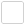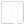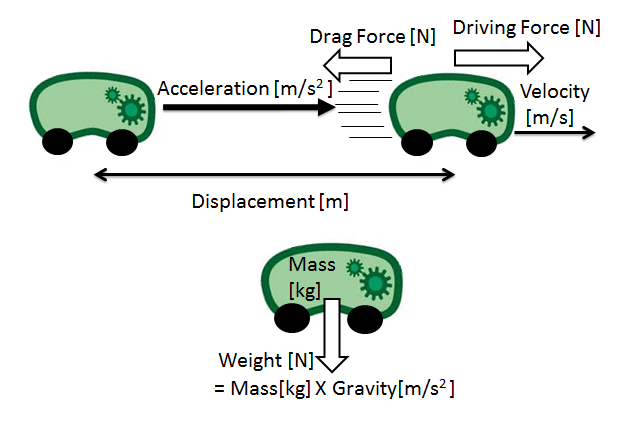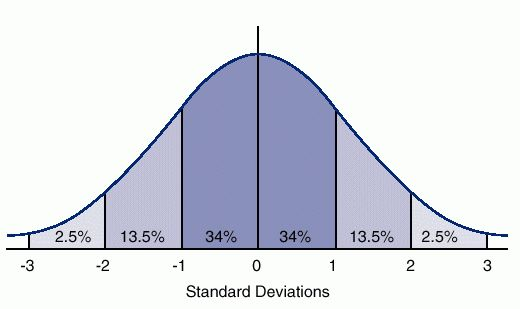# Team:Glasgow/Modeling Intro

Bubble Test Page## Modelling

This section of the wiki deals with the Modelling that the dry lab undertook. As the original aim for the project was to produce floating bacteria, we decided to create a series of models of a bacteria's movement, so as to better understand the limitations of the plan and also to shape its progression.The modelling is largely based on mechanics, so we've prepared a brief introduction to a few of the terms used: feel free to ignore this and head straight to the actual modelling.

Displacement: The net distance travelled by an object. Measured in meters (m).

Velocity: Like speed, but with a specified direction. Measured in metres per second (m/s or ms-1).

Acceleration: How fast the velocity is changing. Measured in meters per second per second (m/s2, ms-2). The acceleration due to gravity on Earth is g, is equal to 9.81ms-2. This is used very frequently.

Force: The “push” or “pull” on an object, which is capable of giving the object a velocity, an acceleration, or changing its shape/deforming it. Units are Newtons (N). When forces are balanced – I,e, an equal push and pull from either side, the object will either be stationary, or be moving with a constant velocity. If they are unbalanced, the object will be accelerating.Stokes drag is a force that applies to small spherical objects in a continuous, viscous fluid. It is somewhat equivalent to air resistance in air, acting opposite to the direction of motion. This particular force is key to the modelling of the system.

Mass: NOT the same as weight! Mass is measuring in kg, while weight is in fact a force – mass*gravitational acceleration.

Density: The mass per every unit of volume (kg/m3). A very dense object has a lot of mass in a very small space – such as lead or a black hole.

Buoyancy: Buoyancy is the principal on which the gas vesicles make the E.Coli float. They work to reduce the density of the bacteria below that of water.
Buoyancy Force = (density of water*volume of object*g) – (density of object*volume of object*g)

Normal DistributionThis is a very important concept in probability theory. It describes one way in which multiple data readings will distribute themselves about a mean value. Its width is determined by the standard deviation. The % values are the % of results that will fall within that number of standard deviation from the mean. For instance 68% of values are within 1 standard deviation of the mean while 95% are within 2 standard deviations from the mean.Random Walk: The random walk is a very common model, often used to describe the movement of gas particles.

Graph of Normal Distribution obtained from: http://medical-dictionary.thefreedictionary.com/normal+distribution
Edit